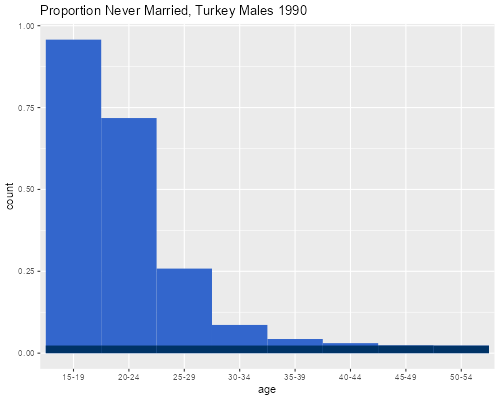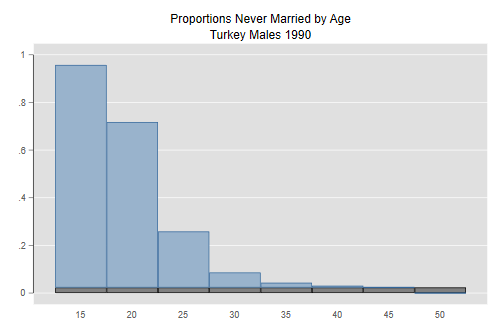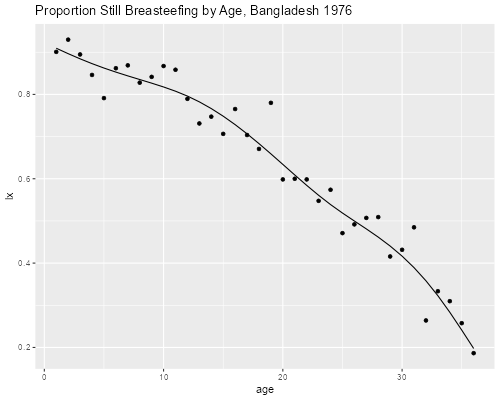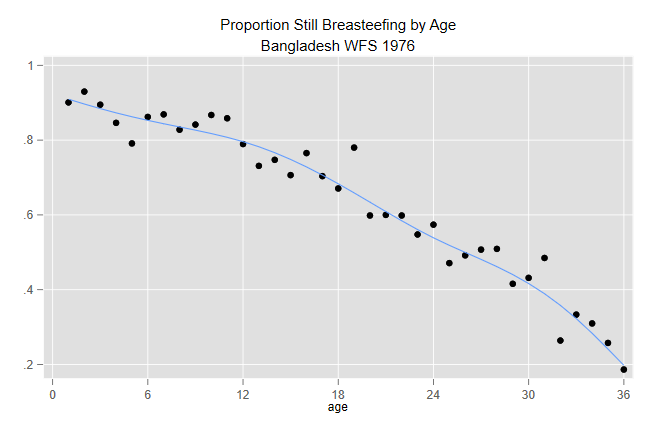Germán Rodríguez
Demographic Methods Princeton University## Current Status Life Tables

We will consider applications of current status life tables to nuptiality and breastfeeding.

### Proportions Married

This is the example on page 90 of the textbook, showing proportions single by age for Turkish men in 1990. I start at age 15 rather than 0 because the proportion ever married is zero in the younger age groups.

```> tk <- read.table("https://grodri.github.io/datasets/turkey90.dat",
+     header = TRUE) # all columns have names
```
```. clear

. import delimited using https://grodri.github.io/datasets/turkey90.dat, ///
>   delim(" ", collapse) clear
(encoding automatically selected: ISO-8859-1)
(3 vars, 8 obs)
```

Next I compute the proportion single in each age group, estimate the proportion single at age 50 `(s50)` as the average of age groups 45-49 and 50-54, and compute SMAM. I add 15 because that’s the age at which I started, and multiply `s50` by 35 (which is 50 - 15).

```. gen p = single/n

. scalar s50 = (p + p)/2

. quietly sum p in 1/7         // exclude 50+

. scalar smam = 15 + (5 * r(sum) - 35 * s50)/(1 - s50)

. display smam
25.003883
```
```> library(dplyr)
> tk <- mutate(tk,
+   age = factor(age, labels=paste(seq(15,50,5),"-",seq(19,54,5),sep="")),
+   p = single/n)
> s <- summarize(tk, p50 = sum(p[7:8])/2,
+   smam =  15 + (5 * sum(p[1:7]) - 35 * p50)/(1 - p50))
> s
p50     smam
1 0.02331215 25.00388
```

SMAM is 25 years and corresponds to the lighter area in the figure below scaled by `1 - s50.` The darker area shows the proportion that will remain single by age 50.

```> library(ggplot2)
> tk <- mutate(tk, s = ifelse( p > s\$p50, s\$p50, p))
> ggplot(tk, aes(age, weight = p)) + geom_bar(width = 1, fill = "#3366cc") +
+   geom_bar(aes(weight = s), width = 1, fill = "#003366") +
+   ggtitle("Proportion Never Married, Turkey Males 1990")
> ggsave("smamtk90r.png", width = 500/72, height = 400/72, dpi = 72)
``````. gen p50 = s50

. gen pr = p - p50

. graph bar p50 pr, stack over(a, gap(0) ) legend(off) ///
>   bar(2, color(51 102 153)) ///
>   title(Proportions Never Married by Age) ///
>   subtitle(Turkey Males 1990)

. graph export smamtk90.png, width(500) replace
file smamtk90.png saved as PNG format
```SMAM is often interpreted as the mean age at marriage conditional on marrying by age 50. This interpretation applies to real cohorts only if we are willing to assume that nuptiality has been constant over the last 35 years. However, the measure is a useful synthetic summary of period nuptiality, even without the assumption of stationarity. (In fact, the unscaled version has a direct interpretation as time lived in the single state by Turkish men in 1990.)

### Duration of Breastfeeding

In an earlier handout on smoothing I showed that retrospective reports of duration of breastfeeding in Bangladesh (as elsewhere), show very substantial heaping at multiples of 12 (and to a lesser extent 6) months. We now use a current status life table to obtain more reliable estimates.

I extracted data from the Bangladesh WFS for all births in the three years preceding the survey and tabulated the number still breastfeeding and the total number of births by current age and type of place of residence (urban, rural). We will collapse the data by age, effectively summing over place of residence.

```> library(haven)
> bd <- group_by(bd, age) |> summarize(stillbr = sum(stillbr), n = sum(n))
```
```. use https://grodri.github.io/datasets/bdbrfeed.dta, clear
(BDSR03 extract)

. collapse (sum) stillbr (sum) n, by(age)
```

The current status estimate of the life table is simply the proportion still breastfeeding at each age:

```> library(dplyr)
> library(ggplot2)
> bd <- mutate(bd, lx = stillbr/n)
> ggplot(bd, aes(age, lx)) + geom_point()
```
```. gen lx = stillbr/n

. scatter lx age
```

A slight problem with this “lx” function (shown below) is that it is not monotone. There is an algorithm to force monotonicity known as “pool adjacent violators”; essentially you use the observed proportions as long as they are non increasing, when you find a violation of monotonicity you backtrack, combining adjacent ages, until the problem dissappears, at which you resume working forward.

A simpler approach is to use a smoother, and the figure below shows a regression spline. I fitted it used logistic regression to take into account the fact that the underlying data are binary, but otherwise the basic idea is the same as before. I use a regression spline with internal knots at 12 and 24 months.

```> library(splines)
> sf <- glm( cbind(stillbr, n - stillbr) ~ bs(age, knots=c(12,24)),
+   data = bd, family = binomial)
> bd <- mutate(bd, fit = fitted(sf))  # default is proportion
> ggplot(bd, aes(age, lx)) + geom_point() + geom_line(aes(age, fit)) +
+   ggtitle("Proportion Still Breasteefing by Age, Bangladesh 1976")
> ggsave("bdbrfeedr.png", width = 500/72, height = 400/72, dpi = 72)
``````. bspline ,xvar(age) knots(0 12 24 36) p(3) gen(bs)

. quietly blogit still n bs*, noconstant

. predict fit, pr

. twoway scatter lx age || line fit age, legend(off) ///
>   xlabels(0 6 12 18 24 30 36) ///
>   title(Proportion Still Breasteefing by Age) ///

. graph export bdbrfeed.png, replace
file bdbrfeed.png saved as PNG format
```An important point to note is that there is no evidence of precipitous declines in proportions still breastfeeding after 12 or 24 months, so the heaping of retrospective reports on these values was probably bad data rather than a real phenomenon. This is why current status life tables are the method of choice for duration data on breastfeeding, post-partum amenorrhea, and post-partum abstinence, which are usually poorly reported.

It is clear that upwards of 90% of all children are breastfed; the exact value is hard to determine because our first data point is at exact age one month, but a direct question suggests it is probably of the order of 95%.

Note also that almost 20% of the kids are still breastfeeding at age 3 exact years. The time spent breastfeeding in the first three years, computed as the area under the “lx” curve, is

```> summarize(bd, Lx = sum(lx), Fit = sum(fit))
# A tibble: 1 × 2
Lx   Fit
<dbl> <dbl>
1  23.0  22.8
```
```. quietly sum lx

. di r(sum)
22.963591

. quietly sum fit

. di r(sum)
22.817572
```

or almost 23 months. Using the spline gives 22.8 months. The mean is probably higher, depending on how far the 20% upper tail extends.

The incidence-prevalence method estimates duration in a state (such as an illness) as a ratio of prevalence to incidence, or existing to new cases. To obtain this estimate we compute the overall proportion still breasteefing at durations 1-36 and divide by 0.95/36, an estimate of cases who start breastfeeding each month:

```> summarize(bd, ipe = weighted.mean(lx, n)/(0.95/36))
# A tibble: 1 × 1
ipe
<dbl>
1  23.8
```
```. summ lx [fw=n]

Variable │        Obs        Mean    Std. dev.       Min        Max
─────────────┼─────────────────────────────────────────────────────────
lx │      3,850    .6275325    .2118189   .1864407   .9298246

. di r(mean)/(0.95/36)
23.780178
```

The incidence-prevalence estimate is 23.8 months, and is consistent with the other results.

Note in closing that with current status data all observations are censored,

• Children still breastfeeding are right censored at their current age (all we know is that they will breastfeed longer than that), and

• Children weaned, or who never breastfed, are left censored at their current age (all we know is that they breastfed less than that).

Yet we can still estimate a survival distribution!

